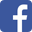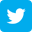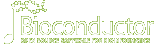# bigPintDOI: 10.18129/B9.bioc.bigPintThis is the development version of bigPint; for the stable release version, see bigPint.

## Big multivariate data plotted interactively

Bioconductor version: Development (3.16)

Methods for visualizing large multivariate datasets using static and interactive scatterplot matrices, parallel coordinate plots, volcano plots, and litre plots. Includes examples for visualizing RNA-sequencing datasets and differentially expressed genes.

Author: Lindsay Rutter [aut, cre], Dianne Cook [aut]

Maintainer: Lindsay Rutter <lindsayannerutter at gmail.com>

Citation (from within R, enter `citation("bigPint")`):

### Installation

To install this package, start R (version "4.2") and enter:

```if (!require("BiocManager", quietly = TRUE))
install.packages("BiocManager")

# The following initializes usage of Bioc devel
BiocManager::install(version='devel')

BiocManager::install("bigPint")```

For older versions of R, please refer to the appropriate Bioconductor release.

### Documentation

To view documentation for the version of this package installed in your system, start R and enter:

`browseVignettes("bigPint")`

 HTML R Script bigPint Vignette HTML R Script Data metrics object HTML R Script Recommended RNA-seq pipeline PDF Reference Manual

### Details

 biocViews Clustering, DataImport, DifferentialExpression, GeneExpression, MultipleComparison, Normalization, Preprocessing, QualityControl, RNASeq, Sequencing, Software, Transcription, Visualization Version 1.13.0 In Bioconductor since BioC 3.9 (R-3.6) (3 years) License GPL-3 Depends R (>= 3.6.0) Imports DelayedArray(>= 0.12.2), dplyr (>= 0.7.2), GGally (>= 1.3.2), ggplot2 (>= 2.2.1), graphics (>= 3.5.0), grDevices (>= 3.5.0), grid (>= 3.5.0), gridExtra (>= 2.3), hexbin (>= 1.27.1), Hmisc (>= 4.0.3), htmlwidgets (>= 0.9), methods (>= 3.5.2), plotly (>= 4.7.1), plyr (>= 1.8.4), RColorBrewer (>= 1.1.2), reshape (>= 0.8.7), shiny (>= 1.0.5), shinycssloaders (>= 0.2.0), shinydashboard (>= 0.6.1), stats (>= 3.5.0), stringr (>= 1.3.1), SummarizedExperiment(>= 1.16.1), tidyr (>= 0.7.0), utils (>= 3.5.0) LinkingTo Suggests BiocGenerics(>= 0.29.1), data.table (>= 1.11.8), EDASeq(>= 2.14.0), edgeR(>= 3.22.2), gtools (>= 3.5.0), knitr (>= 1.13), matrixStats (>= 0.53.1), rmarkdown (>= 1.10), roxygen2 (>= 3.0.0), RUnit (>= 0.4.32), tibble (>= 1.4.2) SystemRequirements Enhances URL https://github.com/lindsayrutter/bigPint BugReports https://github.com/lindsayrutter/bigPint/issues Depends On Me Imports Me Suggests Me Links To Me Build Report

### Package Archives

 Source Package bigPint_1.13.0.tar.gz Windows Binary bigPint_1.13.0.zip macOS 10.13 (High Sierra) Source Repository git clone https://git.bioconductor.org/packages/bigPint Source Repository (Developer Access) git clone git@git.bioconductor.org:packages/bigPint Package Short Url https://bioconductor.org/packages/bigPint/ Package Downloads Report Download StatsDocumentation »

Bioconductor

R / CRAN packages and documentation